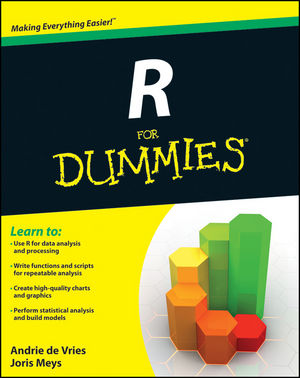## optimising accept-reject

Posted in R, Statistics, University life with tags , , , , , , on November 21, 2012 by xi'an

I spotted on R-bloggers a post discussing optimising the efficiency of programming accept-reject algorithms. While it is about SAS programming, and apparently supported by the SAS company, there are two interesting features with this discussion. The first one is about avoiding the dreaded loop in accept-reject algorithms. For instance, taking the case of the truncated-at-one Poisson distribution, the code

rtpois=function(n,lambda){
sampl=c()
while (length(sampl)<n){
x=rpois(1,lambda)
if (x!=0) sampl=c(sampl,x)}
return(sampl)
}


is favoured by my R course students but highly inefficient:

> system.time(rtpois(10^5,.5))
user  system elapsed
61.600  27.781  98.727


both for the stepwise increase in the size of the vector and for the loop. For instance, defining the vector sampl first requires a tenth of the above time (note the switch from 10⁵ to 10⁶):

> system.time(rtpois(10^6,.5))
user  system elapsed
54.155   0.200  62.635


As discussed by the author of the post, a more efficient programming should aim at avoiding the loop by predicting the number of proposals necessary to accept a given number of values. Since the bound M used in accept-reject algorithms is also the expected number of attempts for one acceptance, one should start with something around Mn proposed values. (Assuming of course all densities are properly normalised.) For instance, in the case of the truncated-at-one Poisson distribution based on proposals from the regular Poisson, the bound is 1/1-e. A first implementation of this principle is to build the sample via a few loops:

rtpois=function(n,lambda){
propal=rpois(ceiling(n/(1-exp(-lambda))),lambda)
propal=propal[propal>0]
n0=length(propal)
if (n0>=n)
return(propal[1:n])
else return(c(propal,rtpois(n-n0,lambda)))
}


with a higher efficiency:

> system.time(rtpois(10^6,.5))
user  system elapsed
0.816   0.092   0.928


Replacing the expectation with an upper bound using the variance of the negative binomial distribution does not make a significant dent in the computing time

rtpois=function(n,lambda){
M=1/(1-exp(-lambda))
propal=rpois(ceiling(M*(n+2*sqrt(n/M)/(M-1))),lambda)
propal=propal[propal>0]
n0=length(propal)
if (n0>=n)
return(propal[1:n])
else return(c(propal,rtpois(n-n0,lambda)))}


since we get

> system.time(rtpois(10^6,.5))
user  system elapsed
0.824   0.048   0.877


The second point about this Poisson example is that simulating a distribution with a restricted support using another distribution with a larger support is quite inefficient. Especially when λ goes to zero By comparison, using a Poisson proposal with parameter μ and translating it by 1 may bring a considerable improvement: without getting into the gory details, it can be shown that the optimal value of μ (in terms of maximal acceptance probability) is λ and that the corresponding probability of acceptance is$\dfrac{1-\exp\{-\lambda\}}{\lambda}$

which is larger than the probability of the original approach when λ is less than one. As shown by the graph below, this allows for a lower bound in the probability of acceptance that remains tolerable.## R for dummies

Posted in Books, R, Statistics, University life with tags , , , , , , , , on October 20, 2012 by xi'anJust saw this nice review of R for dummies. And thought after this afternoon class that my students in the simulation course at Paris-Dauphine could clearly benefit from reading it! They in fact had a terrible time simulating a truncated normal distribution by accept-reject. As they could not get the notion of normalising constants… (Yes, indeed, this very truncated normal distribution!) Even the validity of simulating a normal variate until the truncation is satisfied was not obvious to them and they took forever to program the corresponding code. Anyway, I will certainly order the book to check for myself (after receiving Genetics for dummies to make sure I use the right vocabulary, even though it is a bit too light in the end…)! And write a review for CHANCE if it generates enough interest in doing so…

## simulation, a ubiquitous tool

Posted in pictures, R, Statistics, Travel, University life with tags , , , , , on July 10, 2012 by xi'an

After struggling for quite a while on that AMSI public lecture talk, and dreading its loss with the problematic Macbook, I managed to complete a first draft last night in Adelaide, downloading [at high financial cost!] a final set of images from the Web (plus a few personal ones, like a picture of my son’s Warhammer figurines!). Having very few inklings about the level and the expectations of the audience (if any!), I cannot say if this introduction to simulation is too basic. I will see after the first talk whether or not the aim was off-target… In any case, I am glad I was forced into writing this talk as I had always wanted to have a general-audience introduction to simulation at the ready and can now recycle it easily when and if needed. I will certainly use it in my R class this semester.

## Introducing Monte Carlo in PaRis

Posted in Books, R, Statistics, University life with tags , , , , on November 15, 2010 by xi'an

As already announced on Statisfaction, I will start a short [14 hour] course in English based on Introducing Monte Carlo Methods with R at ENSAE next Tuesday. The slides were written by George Casella for a course he gave in Italy last spring and he kindly agreed on making them available on slideshare: